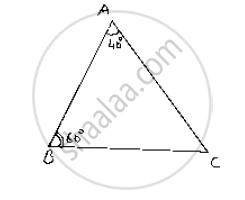# In δAbc, If ∠A = 40° and ∠B = 60°. Determine the Longest and Shortest Sides of The Triangle. - Mathematics

In ΔABC, if ∠A = 40° and ∠B = 60°. Determine the longest and shortest sides of the triangle.

#### Solution

Given that in , ΔABC  ∠A =40°  and ∠B=60° We have to find longest and shortest side We know that,

Sum of angles in a triangle180°

⇒∠A+∠B+∠C=180°

⇒40°+60°+∠C=180°

⇒∠C=180° -(40° +60° )

=180° -100° =80°

∴∠C = 80°

Now,

⇒ 40<60<80⇒∠A< ∠B < ∠C

⇒ ∠Cis greater angle and ∠A is smaller angle

Now, ∠A<∠B<∠C

⇒ BC<AAC<AB

[∵Side opposite to greater angle is larger and side opposite to smaller angle is smaller]

∴ AB is longest and BC is smallest or shortest side.Concept: Properties of a Triangle
Is there an error in this question or solution?

#### APPEARS IN

RD Sharma Mathematics for Class 9
Chapter 12 Congruent Triangles
Exercise 12.6 | Q 1 | Page 81

Share